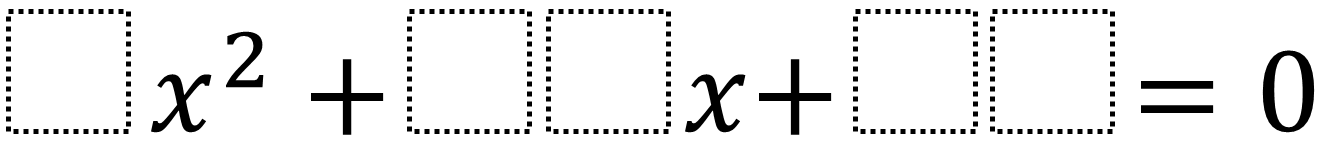Home > High School: Algebra > Factoring Quadratics 2 – One Solution

# Factoring Quadratics 2 – One Solution

Directions: Using the digits 0 to 9 at most one time each, fill in the boxes to so that there is only one integer solution. Note that you can use a 05 to make a 5.### Hint

How could you find the equation if you already knew the solutions?

### Answer

The possible answers are: 4x^2 + 12x + 09, 4x^2 + 36x + 81, 9x^2 + 12x + 04, 9x^2 + 24x + 16, 9x^2 + 30x + 25, 9x^2 + 54x + 81

Source: Daniel Luevanos

## Writing Equivalent Polynomial Expressions

Directions: Use the digits 1-9, at most one time each, to create a true statement. …

### 2 comments

1.Should this say “so that there is only one solution” instead of “so that the solutions are integers”?

•Thanks Michael. We’ve updated the problem. Let us know if there are any other issues.